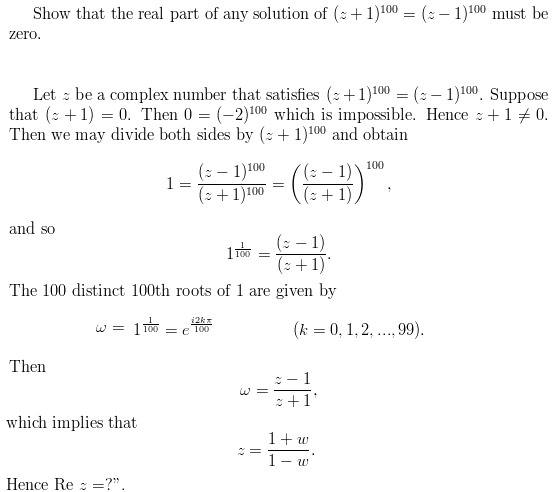# Elementary algebra of complex variables problem

jdinatale
I'm having difficulty deducing that Re z = 0.Staff Emeritus
Homework Helper
Try multiplying the top and bottom by (1-w*).

jdinatale
Try multiplying the top and bottom by (1-w*).

Thank you, that seems to work. Can you confirm that this is correct?

http://i45.tinypic.com/2wc2xl4.png

Last edited by a moderator:
Staff Emeritus
Homework Helper
You made a minor error in calculating the denominator when you canceled the ones.

skiller
Sorry for butting in, but although I can see where jdinatale went wrong in his last post, I still can't see how Re(z) can be proved to be zero.

Of course it's correct, but I can't quite see it.

Homework Helper
Sorry for butting in, but although I can see where jdinatale went wrong in his last post, I still can't see how Re(z) can be proved to be zero.

Of course it's correct, but I can't quite see it.

The calculation shows z is pure imaginary and that's still true after you make the correction. Doesn't that show Re(z)=0?

skiller
The calculation shows z is pure imaginary
How does it?

Sorry, I'm probably being very thick here and will live to regret it.

How does

$$z = (1 + \omega) / (1 - \omega)$$
where $\omega = e^{ik\pi/50}$

imply that $z$ is purely imaginary?

Like I say, I know it's correct to say so, but I'm lost on how to prove it - even after trying using the conjugate method as offered above.

It'll probably be a face-palm moment when I find out...

Homework Helper
How does it?

Sorry, I'm probably being very thick here and will live to regret it.

How does

$$z = (1 + \omega) / (1 - \omega)$$
where $\omega = e^{ik\pi/50}$

imply that $z$ is purely imaginary?

Like I say, I know it's correct to say so, but I'm lost on how to prove it - even after trying using the conjugate method as offered above.

It'll probably be a face-palm moment when I find out...

Look at what happens when jdinatale multiplies by (1+w*). The results has w-w* (which is pure imaginary) in the numerator and w+w* (which is pure real) in the denominator. What kind of a number is imaginary/real?

skiller
Look at what happens when jdinatale multiplies by (1+w*). The results has w-w* (which is pure imaginary) in the numerator and w+w* (which is pure real) in the denominator. What kind of a number is imaginary/real?
Ha-ha! I was right!!!

A complete face-palm...

Thanks for that. I blame my incompetence on my getting on a bit.